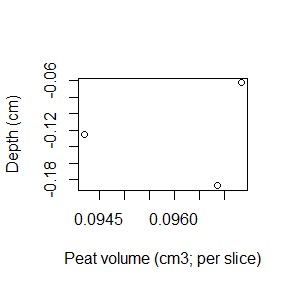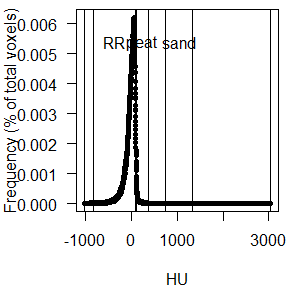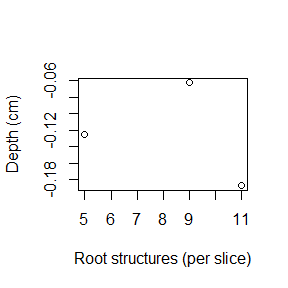# Using coreCT

## Introduction

Computed Tomography (CT) imaging has many applications outside the medical field. One such application in the field of environmental science is scanning sediment cores from coastal wetlands, a technique first demonstrated by Davey et al. (2011)1. The general goal is typically to quantify various soil/sediment components (particles, sand, water, roots) based on their densities; see sources citing Davey et al. (2011) for specific applications.

CT scanning produces large matrices of metadata-rich files with the .dcm extension. The extension is derived from data standard used in CT scanning, called Digital Imaging and Communications in Medicine (DICOM). The coreCT package is a small but powerful set of functions designed to streamline analysis of CT-scanned sediment cores, enabling rapid programmatic processing and synthesis of semi-processed DICOM images. ‘Semi-processed’ means that irrelevant parts of the images (PVC core tubes, etc.) have been masked out, and the only remaining data are for the actual sediment. coreCT builds on functionality in other packages, particulary the oro.dicom package (Whitcher et al. 2011)2 for reading DICOM images and spatial analysis tools in the raster and igraph packages.

Key features of coreCT include:

• An integrated workflow, from conversion of DICOM files to image analysis
• Internal calculation of the abundance (area, volume, wet mass) of seven sediment components: air, live roots and rhizomes, water, peat, particles, sand, and rock/shell
• Quantification of root characteristics: external surface area, volume, and mass of roots in user-defined size classes

This vignette introduces basic usage of the coreCT functions.

## Data: core_426

The core_426 sample dataset included with coreCT includes three DICOM images from a CT-scanned sediment core. Each image represents a 0.625 mm depth interval, so this dataset is very small and only useful for demonstration purposes.

We’ll use this provided dataset to demonstrate how the basic functions , coreHist, conv, and rootSize work. The latter two functions work with a matrix of DICOM images already in the working environment, but coreCT also includes wrapper functions convDir and rootSizeDir that are more flexible, operating on a directory of raw .dcm files and determining inputs like voxel volumes and slice thicknesses by automatically extracting metadata. These wrapper functions load the DICOM images, extract relevant metadata, and analyze the images for components and root characteristics.

## Basic usage

Using the basic functions requires some initial work to extract metadata and convert the raw values to Hounsfield Units, the standard units for CT output.This can be done more easily with coreCT::convDir, which automatically extracts DICOM metadata associated with pixel dimensions and converts raw values to Hounsfield Units:

materials <- convDir("core_426")

plot(-depth ~ peat.cm3, data = materials, xlab = "Peat volume (cm3; per slice)", ylab = "Depth (cm)")## Examining component distributions

The conversion from Hounsfield Units to particle densities is done using calibration rods of known density analyzed with the sediment core. Reasonable default values are provided for four calibrants following Davey et al. (2011): air, water, colloidal silica, and glass. These values are used to define thresholds between soil components. These thresholds, and the distribution of values in the whole data series, can be visualized and returned by coreHist:

HUfreq <- coreHist("core_426")names(HUfreq)
##  "histData" "splits"
HUfreq\$splits
##     material lower upper
## 1        air -1025  -818
## 2         RR  -818    86
## 3      water    86   114
## 4       peat   114   369
## 5  particles   369   750
## 6       sand   750  1342
## 7 rock_shell  1342  3045

conv uses these calibration data to classify voxels into material classes and then calculate four quantities for each of the seven material classes, for each image: (1) average Hounsfield units, (2) total 2D surface area ($$cm^2$$), (3) volume ($$cm^3$$), and (4) mass (g).

The 2D surface area ($$cm^2$$) is calculated as simply the number of pixels in each class, multiplied by the pixel area. Multiplying 2D surface area by the image thickness provides the volume of each component (reported in $$cm^3$$). The relationship between calibration rod Hounsfield units and particle densities is then applied to estimate component masses, by multiplying each voxel’s bulk density by its volume ($$g.cm^3 * cm^3 = g$$).

## Focusing on roots and rhizomes

Root characteristics are calculated by the rootSize function, which also has a wrapper function rootSizeDir that operates on a directory of raw DICOM images.

By default, root characteristics are calculated when convDir is used, but if root data aren’t of interest or a separate set of DICOM images is being used to quantify roots, time can be saved by including rootData = FALSE as an argument to convDir.

Conversely, root characteristics can be analyzed without quantifying sediment composition. This uses the rootSize function and its metadata-scraping wrapper function, rootSizeDir:

rootChars <- rootSizeDir("core_426", diameter.classes = c(1, 2.5, 10))

plot(-depth ~ structures, data = rootChars, xlab = "Root structures (per slice)", ylab = "Depth (cm)")1. Davey, E., C. Wigand, R. Johnson, K. Sundberg, J. Morris, and C. Roman. 2011. Use of computed tomography imaging for quantifying coarse roots, rhizomes, peat, and particle densities in marsh soils. Ecological Applications 21(6): 2156-2171. (link to manuscript)

2. Whitcher, B., V. J. Schmid and A. Thornton. 2011. Working with the DICOM and NIfTI Data Standards in R. Journal of Statistical Software 44(6): 1-28. (link)